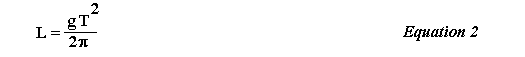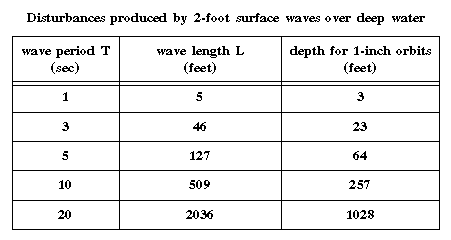# Particle Motion in Deep Water

The small amplitude theory of surface water waves lends itself to a classification of waves based on the relative depth d/L, where d is the water depth and L is the wave length. Waves for which d/L > .5 are referred to as deep water waves. Studies of water particle motions associated with such waves show that, aside from a very small forward displacement (mass transport), the particles beneath a wave essentially move in circular orbits. At the surface the orbit radii are equal in magnitude to the wave amplitude, while for deeper particles the radii decrease exponentially with their distance below the still water surface level (see the figure below).where a is the wave amplitude (as shown).

So, if we know the wave's length and amplitude, we should be able to use Equation 1 to determine how deep we can 'feel' a surface disturbance.